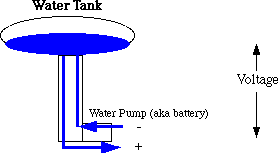# Electricity Tutorial: the Water Model

#### Current and Charge

Think of wires as a pipe and electrical charges (e.g., negatively charged electrons) as water molecules flowing through the pipe.Current is the term used to describe electrical charges moving through a wire. Sort of like the currents and eddies in a creek.

We want to measure the flow of electrical charges over time. Consider counting the number of water molecules moving through a pipe in one second -- a big number!

Coulumb is a unit of charge, consists of 6.3E18 elementary charges (a big number indeed). It is similar to measures like a gallon, i.e., a big aggregation of water molecules. One coulumb per second is defined to be one ampere. Amps are the unit of velocity of electrical flow.

Current (I) = the change in electric charge (dQ) per change in time (dt)

Voltage (V) is the same thing as water pressure: a higher voltage pushes the charges through the wire with greater force. Think of a water tower, with water stored in a tank at the top. The higher the tower, the stronger the water pressure at the bottom of the tower.Power (P) is the rate at which energy changes. It is measured in Watts. Think of a water wheel. The amount of water flowing over the wheel is the current. The height that the water falls is the voltage. Both contribute directly to the generation of power. P = I x V.

Resistance: the thinner the pipe, the higher the resistance, and less water can flow per unit time. The thicker the pipe, the lower the resistance, and more water can flow per unit time. Current, that is electrical flow, is inversely proportional to resistance: V = I x R. Hold voltage fixed, double the resistance, cause the current (flow of electrons) to be halved.

Capacitance: the ability to store charge over time. Like filling a bucket with a hole in the bottom. It takes time to fill ("charge") the bucket ("capacitor"), and over time the charge will leak away. But when the bucket is full, it provides water pressure ("voltage") through the hole.

Inductance: When current flows through a wire, it creates a magnetic field around the wire. Water analogy is like a water wheel attached to a flywheel. A pressure difference -- a voltage -- causes the current to flow initially. Once flowing, the flywheel keeps it flowing even when the voltage is 0.

Last updated by Randy H. Katz, 30 January 1997